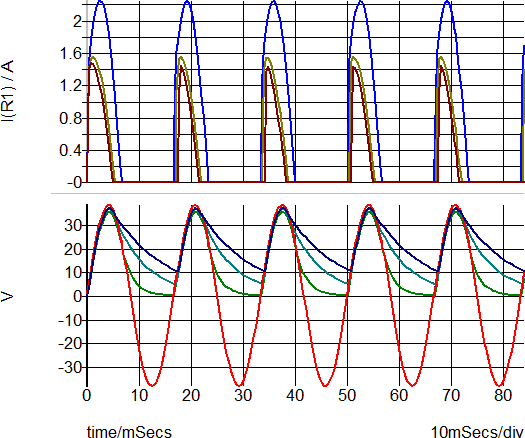# SIMPLIS Multi-step Parameter Analysis

A half-wave rectifier circuit taken from the SIMPLIS Reference Manual provides an example of multi-step parameter analysis. To set up the system to repeat the analysis three times while varying the load resistor, R3, follow these steps:

1. Open the schematic example1.sxsch.
2. To define the value of R3 as an expression relating to a parameter, select and right click on R3 and from the pop-up menu, select Edit/Add Properties.
3. In the Edit Properties dialog box, double click VALUE and change the current value to the following:
`{r3_value}`
Note: You could also use R3.
4. Click Ok twice to return to the schematic.
5. From the menu bar, select Simulator > Setup Multi-step....
6. Enter the following values as shown below:
• Parameter name = r3_value:
• Start value = 20:
• Stop value = 100:
• Number of steps = 37. Click Run.
Result: Three simulations are run using the simulation directives setup defined in the Choose Analysis dialog. Each simulation uses a different resistance value for R3. The resistance values are defined as a linear sweep from 20 to 100 with 3 steps. The waveform viewer opens and displays the output curves:Note: The analysis is repeated three times for the specified values of r3_value: 20, 60 and 100. The resistor value R3 is defined in terms of r3_value which steps R3 through that range.

In most cases, you will step just one part in a similar manner as described above, but you can also use the parameter value to define any number of part values or model values.

If you now run a normal single analysis, you will find that SIMPLIS reports an error because it is unable to resolve the value for R3. This can be overcome by specifying the value using a .VAR control.

8. In the F11 window, add the following line to define the value of R3 when a normal single step analysis is run:
`.VAR r3_value=100 `

## Options

The above example illustrates a linear multi-step parameter run. You can also define a decade (logarithmic) run as well as a list-based run that selects parameter values from a list.

To set up a list run, follow these steps in the Define SIMPLIS Multi-step Analysis dialog box.

1. Select List from the Step type section.
2. Click Define List....
3. Enter the values for the list in the dialog box.
4. Click Run.

The Group Curves check box controls how graphs are displayed.

• If unchecked, curves for each run will have their own legend and curve colour.
• If checked, curves will all have the same colour and share a single legend.